/NumPy 1.17

# numpy.polynomial.legendre.leggauss

`numpy.polynomial.legendre.leggauss(deg)` [source]

Computes the sample points and weights for Gauss-Legendre quadrature. These sample points and weights will correctly integrate polynomials of degree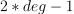or less over the interval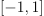with the weight function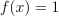.

Parameters: `deg : int` Number of sample points and weights. It must be >= 1. `x : ndarray` 1-D ndarray containing the sample points. `y : ndarray` 1-D ndarray containing the weights.

#### Notes

New in version 1.7.0.

The results have only been tested up to degree 100, higher degrees may be problematic. The weights are determined by using the fact that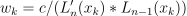whereis a constant independent of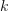and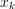is the k’th root of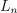, and then scaling the results to get the right value when integrating 1.